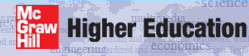# Institut für Thermodynamik der Luft- und Raumfahrt - Universität StuttgartMechanical Engineering - The University of Texas at AustinConvective flows in TEXSTAN are considered an external flow when the pressure gradient is user-supplied from a free-stream velocity distribution that has been derived based on a Mach number distribution, a pressure distribution, or adapted from an Euler solution. The external flow geometries that can be analyzed by TEXSTAN include

• flat plates
• airfoil-shaped surfaces
• axisymmetric converging - diverging nozzles
• two-dimensional converging - diverging nozzles
• bodies of revolution of the missile shape

This section describes how external flows are modeled, and the section boundary layer presents the details of how an external flow dataset is formulated and constructed.

In Reynolds number the x-Reynolds number, momentum thickness Reynolds number, and enthalpy thickness Reynolds number formulations are presented. In friction the surface friction coefficient is formulated. In heat transfer are descriptions of how the surface heat transfer, heat transfer coefficient, and the Nusselt and Stanton numbers are formulated. In mass transfer is an overview of convective mass transfer and descriptions of how the surface mass heat flux and the mass transfer Nusselt and Stanton numbers are formulated.

The integration control subsection describes how the physical and computational domains for an external flow geometry are formulated, how the integration stepsize is controlled, and the concept of entrainment and how it affects integration stepsize.

In initial profiles is a discussion of how TEXSTAN auto-generates laminar, transitional, and turbulent initial (starting) profiles for the various external flow geometries, and how user-supplied initial profiles can be used to start TEXSTAN. In output files is a presentation of the various output options for printing the results of the integration and a description of the files that are formatted to permit easy plotting using an external user-supplied plotting package.

website updated Sept 2010   © 1996-2010 Michael E. Crawford - all rights reserved - website validated for CSS 2.1 and XHTML 1.0 strict at www.w3.org